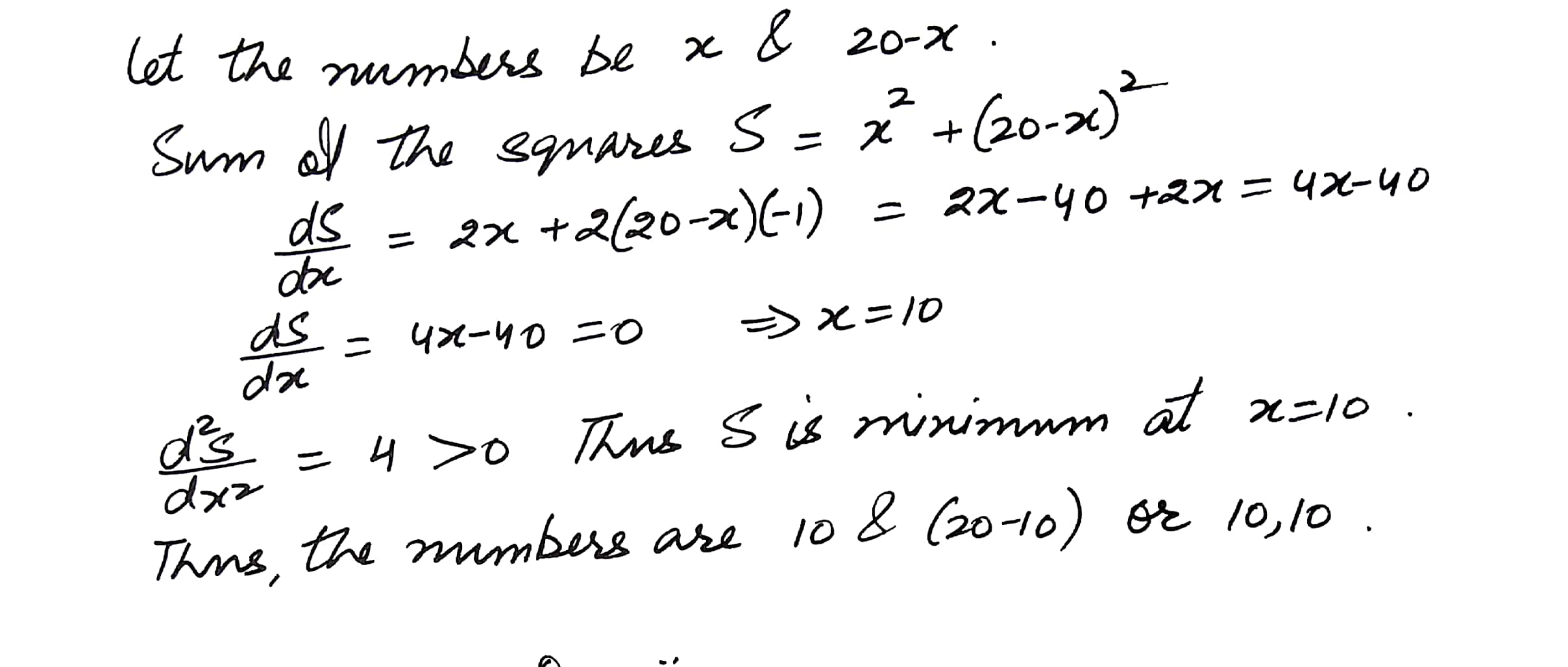5772

Mathematics Integration Level: Misc Level

The sum of two positive numbers is 20. Find the numbers if the sum of their squares is minimum